# How to use the HLOOKUP function in Google SheetsThe HLOOKUP function is perhaps not as famous and widely used as its counterpart, VLOOKUP function. However, the two functions are the same, with the HLOOKUP function simply being the transposed version of VLOOKUP. It stands for ‘Horizontal Lookup’. The function searches for a key value in the first row of the input range, and returns the value of a specified cell from the column where it finds the key. It will produce an error if the key doesn’t exist within the range.

## Syntax

=HLOOKUP(search_key, range, index, [is_sorted])

HLOOKUP(search_key, range, index, [is_sorted])

• search_key – is the value that the HLOOKUP function uses to search.
• range – is the reference to the range of cells that we are performing a search on. Google Sheets application looks for the key in the first row of the range.
• index – this is the row index of the cell within the range, whose value the function returns. The first row’s index within the range is 1, the second in the range is 2 and so on. For example, if we input 3 against this parameter, the HLOOKUP function returns the cell value from the third row and the column in which it finds the search_key.
• [is_sorted] – is an optional parameter which is TRUE by default. This indicates whether the first row in the range is sorted in ascending order or not. If not, we should specify the value as FALSE.

## How to use HLOOKUP function

### Numbers in the first row

Let’s try out a few examples. Here is a test data set with sales figures for a group of salespeople. I will answer various business case questions using HLOOKUP. You’ll see how the index parameter value affects the outcome.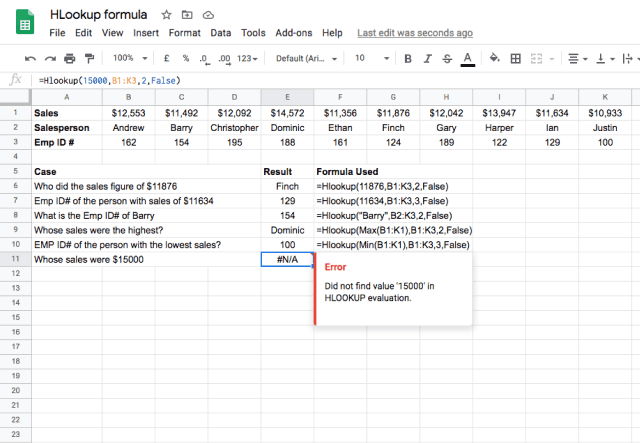I’ll choose first example here to dissect and ensure that you understand the basic use of the function. The formula =Hlookup(11876,B1:K3,2,False) is used to tell the function to search for the value 11,876 within the range of cells from B1 to K3. Once it finds the value, it is instructed to return the data in the second row of the column it found the data in. The False indicates that the data is not sorted, and that you want an exact match to the search key.

Here’s an interesting thing to note in the last case (row # 11 above). The function returned an #N/A error. Why? That is, as explained in the error description, because the \$15000 sales figure does not exist in the first row. Since I specified that I want an exact match (by indicating False for the is_sorted parameter), it cannot return an appropriate value.

### The curious case of [is_sorted]

In the examples, I have chosen FALSE for the last parameter, which tells the function to find an exact match with the search key. This is typically how the HLOOKUP function is used. But what happens when you use True instead to find an approximate match?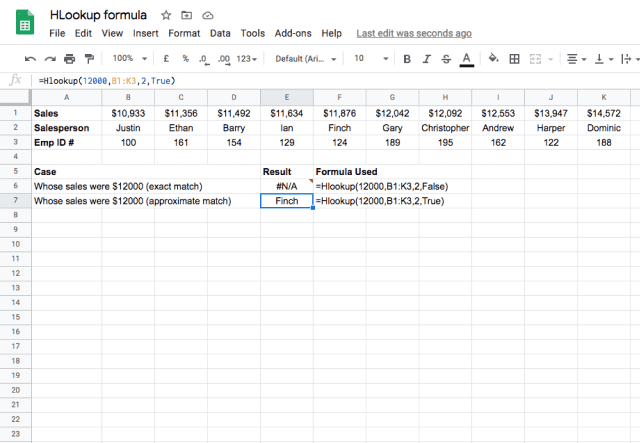Here, there are two examples where we use HLOOKUP to find the person with \$12,000 of sales. The first function uses an is_sorted parameter of False, which tells the function to find an exact match. Because there is no salesperson with exactly \$12,000 of sales, the function returns an error.

The second example is identical except it uses True instead, which tells the function that the data is sorted and it should find an approximate match. The function then tries to find the sales number that is closest to \$12,000, and returns the name of the corresponding salesperson – Finch.

Notice that the salesperson closest to \$12,000 sales is actually Gary. The HLOOKUP function finds an approximate match by finding the value that is closest but not more than the search key. It therefore returns Finch here, even though Gary is technically closer to the search key. This is an important feature to take note of, since it could impact your results when using this function!

Here is a note from the official documentation of the HLOOKUP function:

Using an incorrect sort type may cause incorrect values to be returned.

### Use case: Sorted strings in the first row

Now that you’re getting more familiar with the function, let’s try an example data set in which the first row has sorted string values, as shown below. There are two examples each for all the three cases available, but with one distinction of is_sorted parameter. Please observe how the HLOOKUP function is behaving.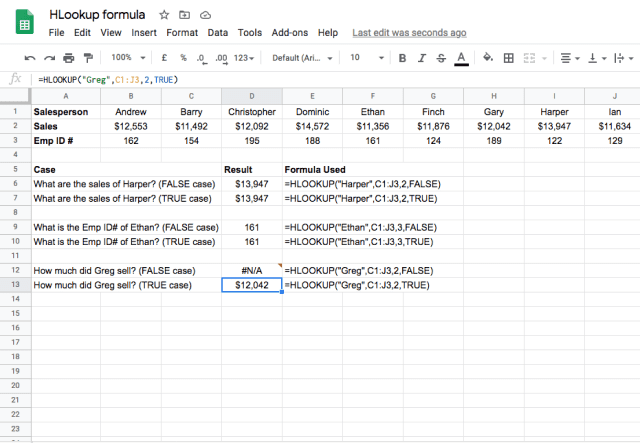You can see that the TRUE or FALSE value for is_sorted parameter doesn’t really affect the behaviour when it finds the search_key within the first row of the range. But an interesting development happens when it doesn’t find an exact match for the search_key. In the case of FALSE (row # 12), it was looking for an exact match. But in the case of TRUE (row # 13), it is looking for an approximate match, and instead of Greg, it returns the information for Gary. This behaviour may or may not be desirable depending on your situation!

### Use case: Unsorted strings in the first row

The data for this case is essentially the same as that used above, except there are non-sorted strings in the first row.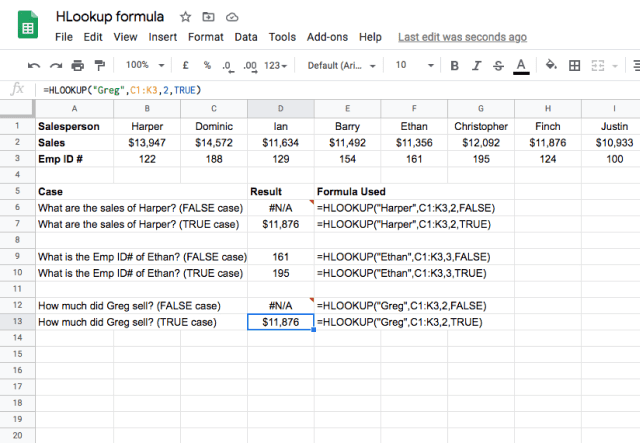Unlike the previous example, the use of TRUE and FALSE values for the fourth parameter is generating different results. Because the data is not sorted, the function cannot give an accurate approximate match. When the data is not sorted, that the results are correct only when I used FALSE for is_sorted.

So, what is the bottom line?

Never lie to Google Sheets in the is_sorted parameter – if you do, it will return the favor!

### Use case: Multiple matches in the first row

There will be times when you may encounter multiple instances of the same value in the first row. In the example below, Barry occurs twice. What happens if you use the HLOOKUP function in such a scenario?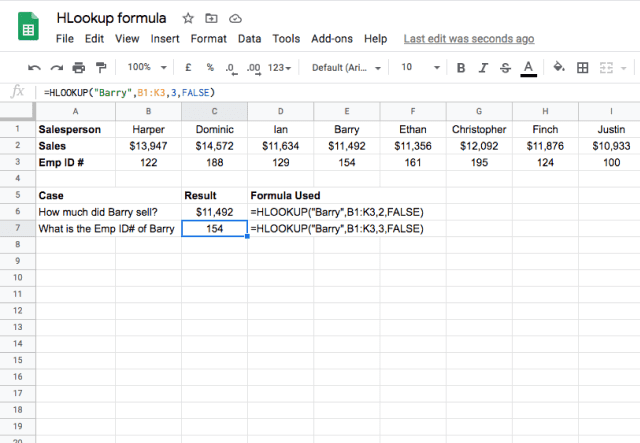You may have noticed, it picks the first Barry it encounters in the list, and doesn’t consider the second Barry.

### Drawbacks with the HLOOKUP function

There are two problems with this function:

1. For looking up search_key, it always uses the first row within the input range-. So it is not possible with the HLOOKUP function to fetch a cell value that is above the lookup row.
2. The function is not dynamic enough, in that, inserting a row between the input range doesn’t automatically update the row index.

There’s an alternative that solves the above two problems. Please go through the explanation for the INDEX and MATCH function combination. It is explained in the context of VLOOKUP function, but is equally applicable as a HLOOKUP alternative.

Editor’s note: This is a revised version of a previous post that has been updated for accuracy and comprehensiveness.